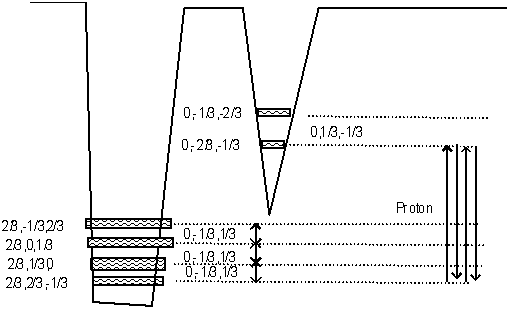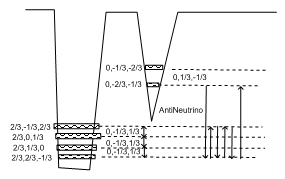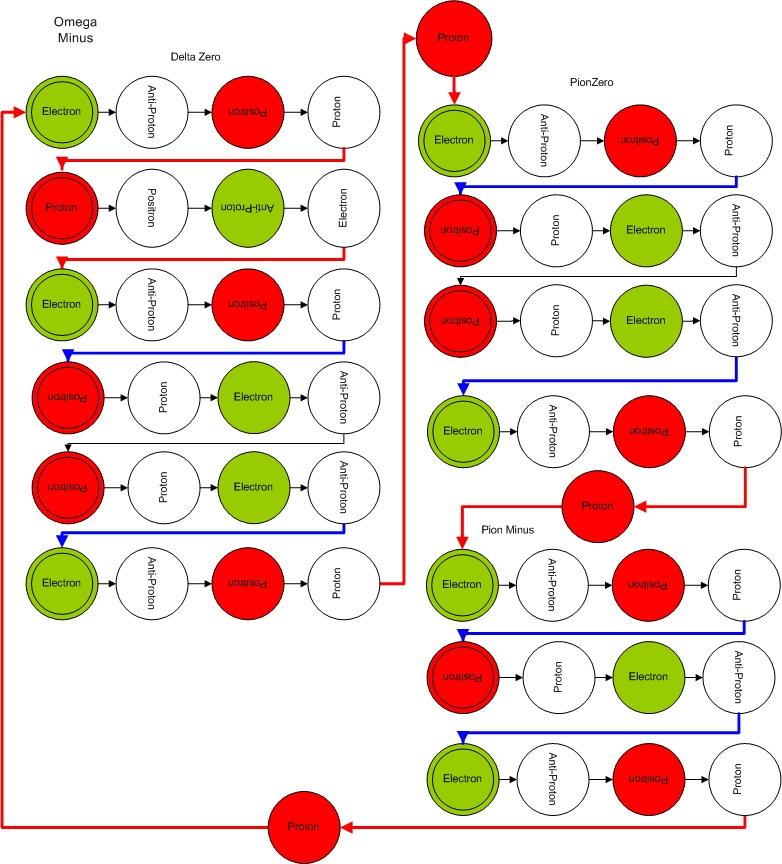#This project is part of the Hypergeometrical Universe Theory. A theory that describes the whole Universe in terms of just space and its metric.

The github repository is:

https://github.com/ny2292000/hypergeometry

The theory contains three parts:
* Cosmogenesis
* Cosmology (or Universe Topology)
* Hypergeometrical Universe Standard Model

## Cosmogenesis

Since everything is made from space and space deformations, Cosmogenesis from Nothing can be achieved. In fact, Nothing is not the best description of the precursor of the Universe. I started with what we observe, an alive (in the sense that it cannot be infinitely precisely defined - Heisenberg Principle) metric.

That metric can start from a zero dimensional metric (like a numerical fluctuation 1 and -1, 2 and -2, 3 and -3, 5 and -5, 7 and -7, 11 and -11... where the sum is always zero). The dimensionality of the metric also can fluctuate from zero dimension, to one dimension (points along a line), to two dimensions( antisymmetric/symmetric deformation in a plane), to three dimensions (antisymmetric/symmetric volume in a cube), to four dimensions (antisymmetric/symmetric hypervolume in a hypercube).

The anti-symmetric or symmetric corresponds to shapes of electronic orbitals 2s and 2p. Negative values of the orbital wavefunction would map to an local stretch of the metric and positive values would map to a compression of the local metric).

An 1s orbital wouldn't be possible since the integral of the compression/stretching wouldn't be zero. Integral of the metric (distance) along line passing through the center of initial fluctuation should result in a Cartesian Distance (if the integral extends over the range of the fluctuation).

The initial fluctuations would break down into smaller pieces and entropy would define how long that Universe would last. In a fourth spatial dimensional Universe, there is spin (rotation around an axis perpendicular to R) in addition to rotation (rotation around the R axis or within the 3D Hypersphere). That together with linear momentum entropy defines the lifetime of this Universe.

Initial Macroscopic Fluctuation decays into a myriad of very, very small coherences (Fundamental Dilator). These coherences recombines creating the initial Gamma Ray explosion that propelled the 3D Universe shell outwards.

Interaction happens through a Quantum Lagrangian Principle that states that each dilator will dilate space in phase with the surrounding dilaton field. This together with equipartition of energy (between radial and tangential degrees of freedom)imposes the lightspeed expansion of the Universe. This is a minimum work or space deformation quantization principle.

## Cosmology

The Universe is proposed to be a 3D Hypersphere traveling at the observed speed of light along the perpendicular direction (Radial Direction). Since this is a moving reference frame, the actual speed of light is deemed to be SQRT(2)*c.

Expansion of the Universe doesn't require Dark Energy and it is explained in pure geometrical terms. The Fourth-Dimensional Radius of the Universe is c*theAgeOfTheUniverse.

Interaction (Natural Forces) is derived from first principles using the Quantum Lagrangian Principle. Gravitation is different from electrostatic interaction in the same way that these two angles (\alpha0 and \alpha1) differ from each other:The radius of the Universe is around 15 Billion light years. The de Broglie step (interaction occurs along the expansion of the Universe in steps equal to the Compton wavelength of a hydrogen atom). That ratio is equal to the ratio between alpha0 and alpha1 and it is also equal to the ratio between Gravitation and Electrostatic Forces.

The rate of change in angle as a function of time (or R) is proportional to acceleration and thus to force. A dilator can move to position x and have its local metric deformed such that it points along alpha1 (electrostatic force) or move to position x and have its metric totally relaxed (pointing along angle alpha0 - gravitation).

## Standard Model

The standard model is based on the proposition that matter is a coherent between two stationary local metric deformational states.
Proton:The assignment of these two states to electron and proton is supported by them being the ground state of each potential well and being eternal. The coherence means that electron, proton, anti-electron(positron) and antiproton are just faces of the same coherence. This eliminates the asymmetry between electron and proton and allows for a simple description of Nature.

The character of a particle is maintained due to the spin. As the coherence changes from state to state, it also spins. If the thickness of the coherence along the R direction is smaller than along the tangential (x,y,z) direction, then when it rotates 90 degrees, it cross section for interaction diminishes, thus creating an Stroboscopic Universe or pseudo-time quantization.

Interaction only occurs when the dilator is flat on the 3D hypersurface, which creates a quantization aspects of this Universe. The other is the spin half, that is the tunneling and spinning frequencies have a half relationship otherwise particles wouldn't "exist" (interaction would vanish due to random averaging). Neutrinos will be described as dilations associated with rotations within the 3D Universe. The dimensionality and levels associated with them makes their frequency different from the Fundamental Dilator and thus interaction is only possible at very close range (small number of strong integration steps).

Now let's see the representation of the Fundamental Dilator. Most matter in the Universe is made of Fundamental Dilator and Polymers of it. Below is the Ball Diagram representation of Electron and Proton.

The spinning lettering maps spinning within the 4D Non-Compact Spatial Manifold.The in-phase initial state is represented within the level diagram below as the initial state:
Electron:The relationship between spinning and tunneling can be modified (transmutation) if one adds a rotation within the 3D Hypersurface (transmutation note). The corresponding metric deformation associated with a 3D rotation on the Proton state (by assignment) corresponds to a half-electron-neutrino and it is represented by the red line linking two fundamental dilators (electron and proton).The other transmutation note is associated with pions where it convert an electron into a positron.The neutron coherence is presented below:and the Balls Diagram:Notice the rotation in the proton state (lower potential well state (2/3,2/3,-1/3) -> (2/3,-1/3,2/3)). This could be a simple rotation around the x axis or be a more complex rotation
(2/3,2/3,-1/3) -> (2/3,-1/3,2/3)-> (-1/3,2/3,2/3)-> (2/3,2/3,-1/3)
This rotation delays spinning (shifting the phase relationship between spinning and tunneling) and changes the in-phase state with respect to the 3D Universe. This is called a transmutation note and in this case it maps into a half-anti-electron-neutrino.

Similarly, rotation in the upper potential well (electron) would give rise to another neutrino (associate with pions) and to a transmutation between electron and positron (PI delay)
By comparison it should be evident the antineutrino coherence below:Given the Fourier decomposition of this coherence being different from anything derived from the Fundamental Dilator Coherence, interaction integrated down to zero unless neutrino and matter collides, thus the Ghost-like nature of neutrinos.

More complex hyperons follow similar logic.

Below is the Omega Hyperon:The initial tasks associated with the coding are:
a) Create classes that can represent themselves as diagrams to illustrate the ideas.
b) Calculate the delay associated with proton rotation in modeling Neutron.
c) Calculate the delay associated with electron rotation in modeling Pion Minus
d) Using observed Moment of Inertia or Magnetic dipole/quadrupole moments, derive the radius of each more complex coherence.

In a complex coherence (many-fundamental-dilator), there is always uncertainty which Fundamental Dilator comes first, this creates a polymer that rotates around the R axis(perpendicular to the 3D Universe). This polymer has a polygonal cross-section. In the case of charged hyperons, it corresponds to polygons with prime number of vertices.

Lifetimes of particles can be mapped to the inverse of total phase mismatch (bandwidth).

Please, feel free to clone the project and give your best shot..:)

Cheers,

MP

Currently unrated

or